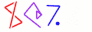Next You can't get there from here 37

# Anything

• This argument works just as well to show that

• We can't recognize functions that add two numbers together

```          my \$newcode = q{sub { my \$f = eval "CODE";
\$f->("INPUT");
return \$_ + \$_;
}};
```
• We can't recognize functions that solve the knapsack problem

```          my \$newcode = q{sub { my \$f = eval "CODE";
\$f->("INPUT");
return solve_knapsack(@_);
}};
```
• We can't recognize functions that will commit invalid array accesses

```          my \$newcode = q{sub { my \$f = eval "CODE";
\$f->("INPUT");
@a = (1..3); \$z = \$a;
}};
```
• Etc.

• Because if we could, we could also recognize functions that halt

• And we can't recognize functions that halt

 NextCopyright © 2005 M. J. Dominus